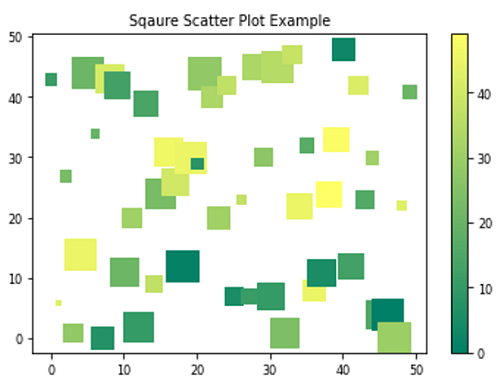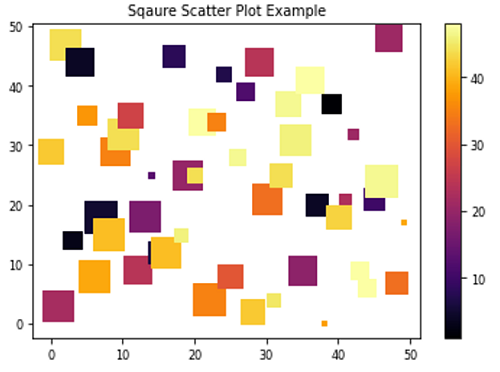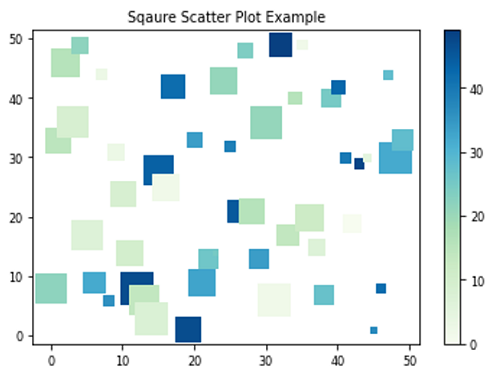# Square Scatter Plot in Python using Matplotlib

In this article, we are going to learn about the square scatter plot in python using matplotlib and its Python implementation.
Submitted by Anuj Singh, on August 14, 2020

Inherited from the Dot Plots, Scatter plots are of very similar types. It provides a power of different features for every individual point. We are allowed to vary the size, color, and other properties of each data point and which makes data more friendly to visualize. Normally, the scatter plot has a circular marker and it could seem generic to anyone. So, instead of using traditional circular markers, we can also use square markers and it also looks good. In this article, we are presenting some random data plotting using a square scatter plot. Moreover, there are three different colormaps for a better understanding of data visualization using a square marker.

Illustrations:## Python code for square scatter plot using matplotlib

```import numpy as np
import matplotlib.pyplot as plt

# Example 1
x = np.arange(50)
y = np.random.randint(0,50,50)
ss = np.random.randint(0,50,50)
c = np.random.randint(0,50,50)

plt.figure()
plt.scatter(x,y, s=ss*10, c=c, marker='s', cmap='summer')
plt.title('Sqaure Scatter Plot Example')
plt.colorbar()

# Example 2
x = np.arange(50)
y = np.random.randint(0,50,50)
ss = np.random.randint(0,50,50)
c = np.random.randint(0,50,50)

plt.figure()
plt.scatter(x,y, s=ss*10, c=c, marker='s')
plt.title('Sqaure Scatter Plot Example')
plt.colorbar()

# Example 3
x = np.arange(50)
y = np.random.randint(0,50,50)
ss = np.random.randint(0,50,50)
c = np.random.randint(0,50,50)

plt.figure()
plt.scatter(x,y, s=ss*10, c=c, marker='s', cmap='GnBu')
plt.title('Sqaure Scatter Plot Example')
plt.colorbar()
```

Output:

```Output is as Figure
```